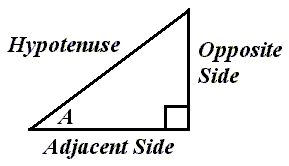# The Right Triangle and the Tangent FunctionRelative to angle A, this is how the sides of a right triangle would be labeled.

The tangent of angle A equals the length of the opposite side divided by the length of the adjacent side.

We could write: